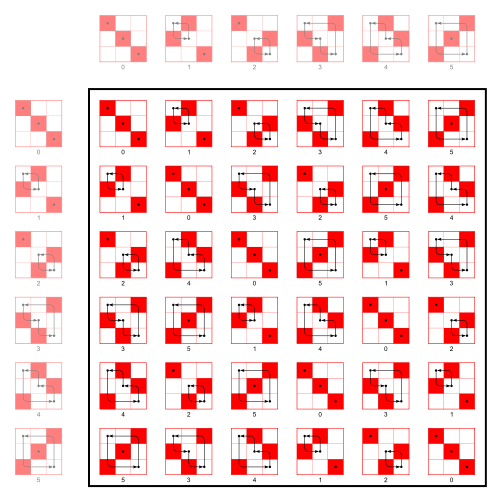This post is devoted to certain properties of random permutation matrices. Let ${\mathfrak{S}_n}$ be the symmetric group, ${n\geq2}$, and let ${\mathcal{P}_n}$ be the group of ${n\times n}$ permutation matrices, obtained by the isomorphism ${\sigma\in\mathfrak{S}_n\mapsto P_\sigma={(\mathbf{1}_{j=\sigma(i)})}_{1\leq i,j\leq n}}$. We know that ${\mathcal{P}_n}$ is a subgroup of the orthogonal group ${\mathbb{O}_n}$. It is also the set of extremal points of the Birkhoff polytope ${\mathcal{B}_n}$ formed by doubly stochastic matrices (these matrices with entries in ${[0,1]}$ with rows and columns all summing to one). The uniform probability measure on ${\mathcal{P}_n}$, which gives mass ${1/n!}$ to every atom, is a Haar measure (left and right translation invariant). We denote by ${J}$ the ${n\times n}$ matrix with all entries equal to ${1}$. We have ${\frac{1}{n}J\in\mathcal{B}_n}$.

Theorem 1 (Moments and fixed points) Let ${k\geq1}$ and ${\psi_n(k):=\mathrm{Card}\{1\leq r\leq n:r \vert k\}}$. If ${P}$ is a random matrix uniformly distributed on ${\mathcal{P}_n}$, and ${F_k:=\mathbb{E}(P^k)}$, then

$\mathrm{diag}(F_k)=\frac{\psi_n(k)}{n}I \quad\text{and in particular}\quad \mathrm{Tr}(F_k)=\psi_n(k).$

If ${\sigma}$ is a random permutation uniformly distributed on ${\mathfrak{S}_n}$, then

$\mathbb{E}(\mathrm{Card}\{1\leq i\leq n:\sigma^k(i)=i\}) =\psi_n(k).$

Note that ${n\mapsto\psi_n(k)}$ grows until ${\psi_k(k)=\mathrm{Card}\{1\leq r\leq k:r\vert k\}}$.

Proof: The second part follows from the first part since if ${P_\sigma:={(\mathbf{1}_{j=\sigma(i)})}_{1\leq i,j\leq n}}$ then ${P^k\overset{d}{=}P_\sigma^k=P_{\sigma^k}}$, and ${\mathrm{Tr}(P_{\sigma^k})=\mathrm{Card}\{1\leq i\leq n:\sigma^k(i)=i\}}$, and thus

$\mathrm{Tr}(F_k) =\mathbb{E}(\mathrm{Tr}(P_{\sigma^k})) =\mathbb{E}(\mathrm{Card}\{1\leq i\leq n:\sigma^k(i)=i\}).$

To establish the first part, we write, for all ${1\leq i\leq n}$,

$(F_k)_{i,i} =\mathbb{E}((P_{\sigma^k})_{i,i}) =\mathbb{E}(\mathbf{1}_{\sigma^k(i)=i}) =\mathbb{P}(\sigma^k(i)=i) =\frac{1}{n!}\sum_{\sigma\in\mathfrak{S}_n}\mathbf{1}_{\sigma^k(i)=i}.$

Now ${i}$ is a fixed point of ${\sigma^k}$ iff ${i}$ belongs to a cycle of ${\sigma}$ of length ${r}$ such that ${r\vert k}$. There are

$A_{n-1,r-1}=\frac{(n-1)!}{(n-1-(r-1))!}=\frac{(n-1)!}{(n-r)!}$

ways to choose this cycle, and ${(n-r)!}$ ways to permute the elements outside this cycle. Therefore

$\frac{1}{n!}\sum_{\sigma\in\mathfrak{S}_n}\mathbf{1}_{\sigma^k(i)=i} =\frac{1}{n!}\sum_{\substack{1\leq r\leq k\\r\vert k}}\frac{(n-1)!}{(n-r)!}(n-r)! =\frac{\psi_n(k)}{n}.$

Theorem 2 (Structure of ${F_k}$) With the settings of theorem 1, we have

$F_k =\frac{\psi_n(k)-1}{n-1}I +\left(1-\frac{\psi_n(k)-1}{n-1}\right)\frac{1}{n}J.$

In particular, the matrix ${F_k}$ belongs to the interval ${[I,\frac{1}{n}J]}$ in ${\mathcal{B}_n}$.

Proof: Let ${\sigma}$ be a random permutation following the uniform distribution on ${\mathfrak{S}_n}$, as in theorem 1. For any fixed ${\tau\in\mathfrak{S}_n}$, we have ${\tau\sigma\overset{d}{=}\sigma\overset{d}{=}\sigma\tau}$, and thus

$P_\tau C_\tau=F_k=C_\tau P_\tau$

where ${C_\tau=\mathbb{E}(P_\sigma\underbrace{P_\tau P_\sigma\cdots P_\tau P_\sigma}_{k-1\text{ times}})}$. Therefore, for any ${\tau\in\mathfrak{S}_n}$,

$P_{\tau^{-1}}F_k=F_kP_{\tau^{-1}}=C_\tau \ \ \ \ \ (1)$

and thus, for any ${\tau\in\mathfrak{S}_n}$

$P_{\tau^{-1}}F_kP_{\tau}=F_k.$

In other words, for any ${\tau\in\mathfrak{S}_n}$ and any ${1\leq i,j\leq n}$,

$(F_k)_{\tau(i),\tau(j)}=(F_k)_{i,j}.$

Thus ${(F_k)_{i,i}=(F_k)_{1,1}}$ and ${(F_k)_{i,j}=(F_k)_{1,2}}$ for any ${1\leq i\neq j\leq n}$. Since ${F_k}$ is a stochastic matrix, we have ${1=F_{1,1}+(n-1)F_{1,2}}$. Now theorem 1 gives ${F_{1,1}=\frac{\psi_n(k)}{n}}$, and the value of ${F_{1,2}}$ follows. ☐

Algebra. For ${k=1}$, the formula (1) allows to determine ${F_1}$ without using theorem 1. Namely, for ${k=1}$, we have ${C_\tau=\mathbb{E}(P_\sigma)=F_1}$, and thus ${P_\tau F_1=F_1=F_1P_\tau}$. Therefore ${F_1}$ has all its rows identical, and all its columns identical, and thus all its entries identical. Since ${F_1}$ is a stochastic matrix, we obtain ${F_1=\frac{1}{n}J}$ and we recover in particular that ${\mathrm{Tr}(F_1)=1=\psi_n(1)}$. For ${k>1}$, the matrix ${C_\tau}$ depends on ${\tau}$, and we ignore how to deduce ${F_k}$ by using simply the translation invariance of the law of ${\sigma}$, without using the combinatorics of the cycles structure of ${\sigma}$.

Blocs and cycles. We may play with the cycles decomposition of ${\sigma}$ using matrices. Namely, we know that ${P_\sigma}$ is conjugated in ${\mathcal{P}_n}$ to ${\oplus_{i=1}^\ell B_i}$ where ${B_1\in\mathcal{P}_{n_1},\ldots,B_\ell\in\mathcal{P}_{n_\ell}}$ are the matrices of the ${\ell}$ cycles of ${\sigma}$, of lengths ${n_1,\ldots,n_\ell}$. Thus ${P_\sigma^k}$ is conjugated to ${\oplus_{i=1}^\ell B_i^k}$. But ${\mathrm{Tr}(B_i^k)=n_i\mathbf{1}_{n_i\vert k}}$ and thus

$\mathrm{Tr}(P_\sigma^k) =\sum_{i=1}^\ell\mathrm{Tr}(B_i^k) =\sum_{i=1}^\ell n_i\mathbf{1}_{n_i\vert k}.$

Denoting ${A_1,\ldots,A_n}$ the number of cycles of ${\sigma}$ of lengths ${1,\ldots,n}$, we get

$\mathrm{Tr}(P_\sigma^k)=\sum_{j=1}^n jA_j\mathbf{1}_{j\vert k}.$

Therefore, theorem 1 gives

$\sum_{j=1}^n j\mathbb{E}(A_j)\mathbf{1}_{j\vert k} =\mathbb{E}(\mathrm{Tr}(P_\sigma^k)) =\psi_n(k).$

Not a surprise for the readers knowing that ${\mathbb{E}(A_j)=\frac{1}{j}}$ for any ${1\leq j\leq n}$.

Note. This post comes from discussions with Yan Doumerc.

This site uses Akismet to reduce spam. Learn how your comment data is processed.

Syntax · Style · .